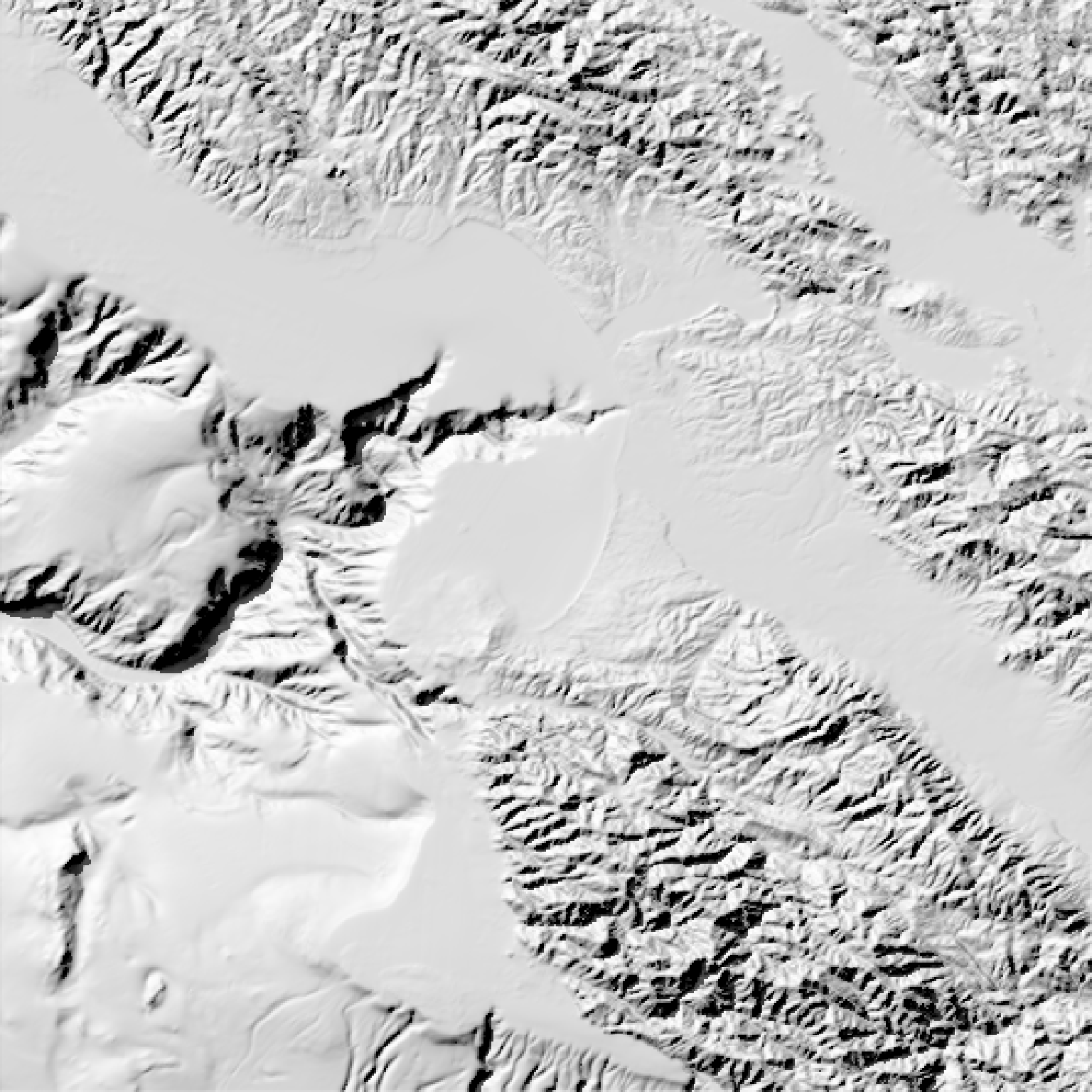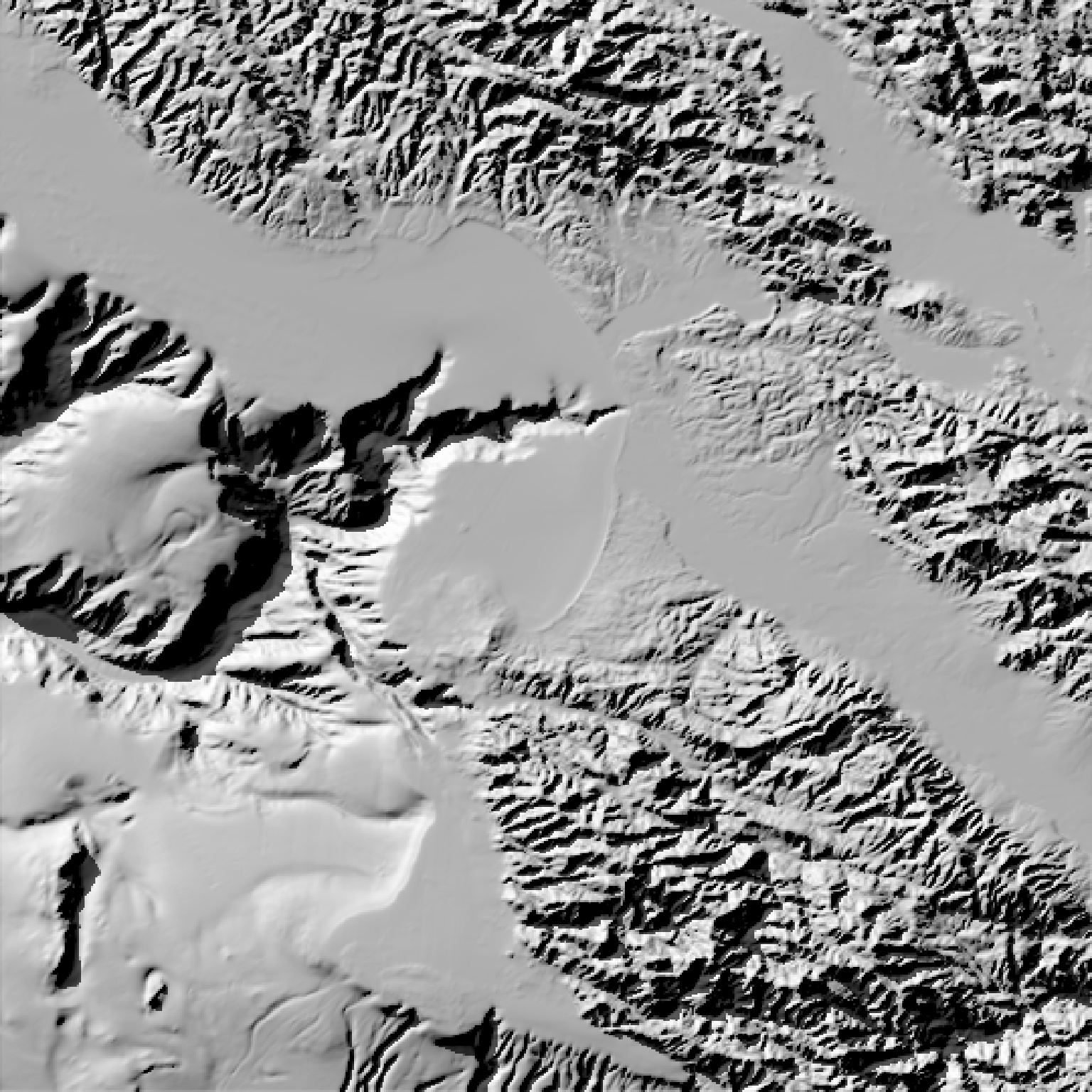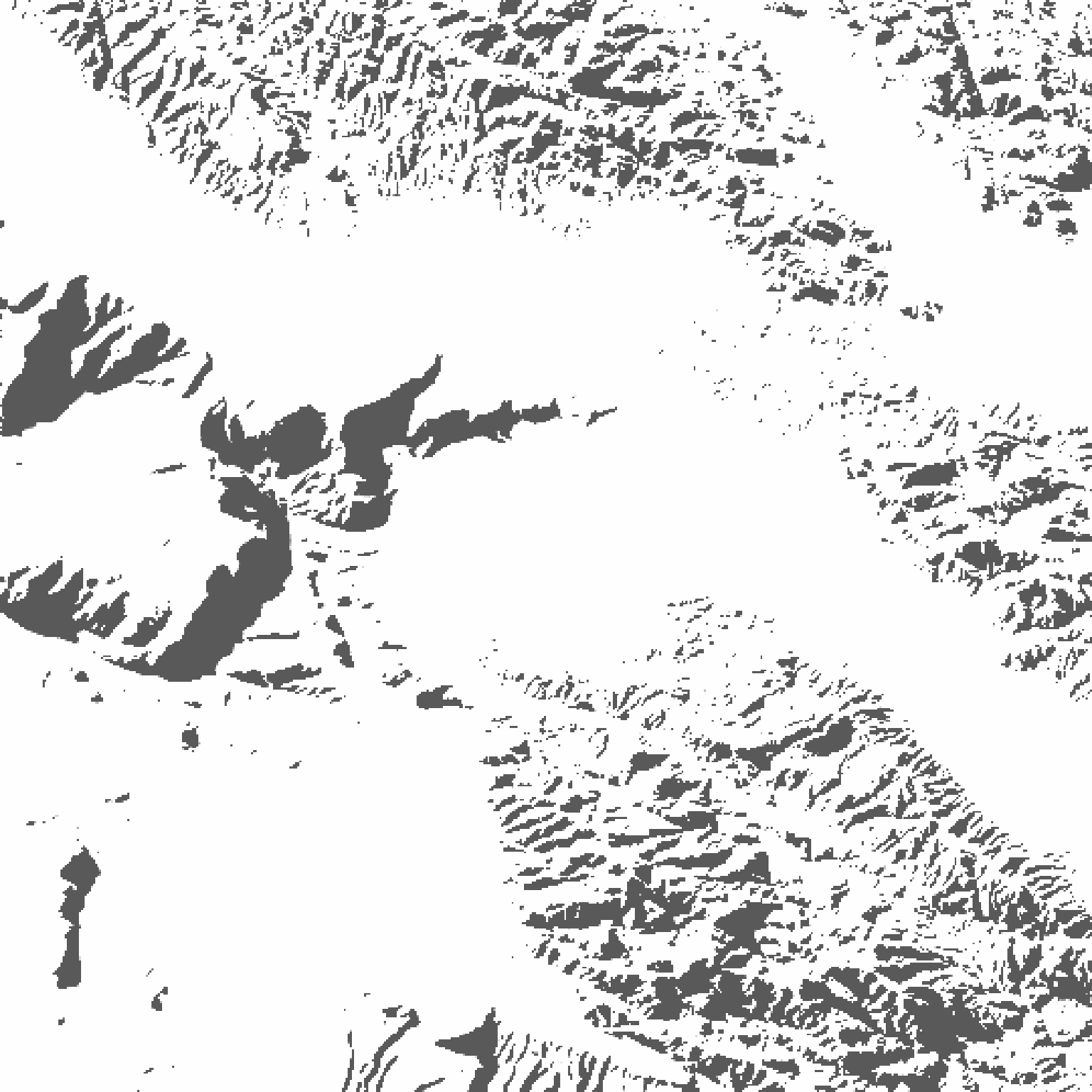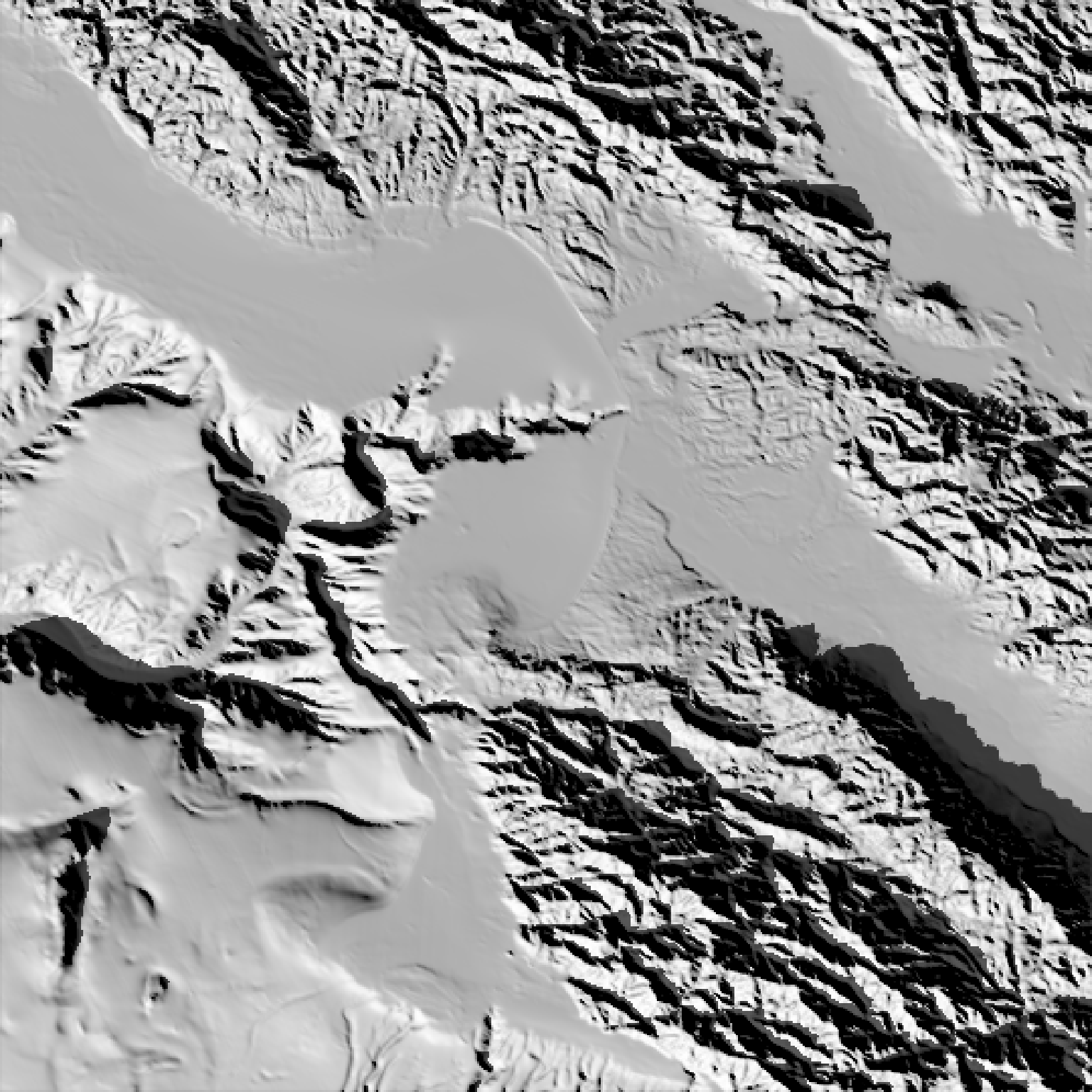Calculates shadow map for a elevation matrix by propogating rays from each matrix point to the light source(s), lowering the brightness at each point for each ray that intersects the surface.

ray_shade(
heightmap,
sunaltitude = 45,
sunangle = 315,
maxsearch = NULL,
lambert = TRUE,
zscale = 1,
multicore = FALSE,
progbar = interactive(),
anglebreaks = NULL,
...
)

## Arguments

heightmap

A two-dimensional matrix, where each entry in the matrix is the elevation at that point. All points are assumed to be evenly spaced.

sunaltitude

Default 45. The angle, in degrees (as measured from the horizon) from which the light originates. The width of the light is centered on this value and has an angular extent of 0.533 degrees, which is the angular extent of the sun. Use the anglebreaks argument to create a softer (wider) light. This has a hard minimum/maximum of 0/90 degrees.

sunangle

Default 315 (NW). The angle, in degrees, around the matrix from which the light originates. Zero degrees is North, increasing clockwise.

maxsearch

Defaults to the longest possible shadow given the sunaltitude and heightmap. Otherwise, this argument specifies the maximum distance that the system should propagate rays to check.

lambert

Default TRUE. Changes the intensity of the light at each point based proportional to the dot product of the ray direction and the surface normal at that point. Zeros out all values directed away from the ray.

zscale

Default 1. The ratio between the x and y spacing (which are assumed to be equal) and the z axis. For example, if the elevation is in units of meters and the grid values are separated by 10 meters, zscale would be 10.

multicore

Default FALSE. If TRUE, multiple cores will be used to compute the shadow matrix. By default, this uses all cores available, unless the user has set options("cores") in which the multicore option will only use that many cores.

Default NULL. A matrix of 1 and 0s, indicating which points on which the raytracer will operate.

Default NULL. The shadow matrix to be updated at the points defined by the argument cache_mask. If present, this will only compute the raytraced shadows for those points with value 1 in the mask.

progbar

Default TRUE if interactive, FALSE otherwise. If FALSE, turns off progress bar.

anglebreaks

Default NULL. A vector of angle(s) in degrees (as measured from the horizon) specifying from where the light originates. Use this instead of sunaltitude to create a softer shadow by specifying a wider light. E.g. anglebreaks = seq(40,50,by=0.5) creates a light 10 degrees wide, as opposed to the default

...

Additional arguments to pass to the makeCluster function when multicore=TRUE.

## Value

Matrix of light intensities at each point.

## Examples

#First we ray trace the Monterey Bay dataset.
#The default angle is from 40-50 degrees azimuth, from the north east.
# \donttest{
montereybay %>%
plot_map()# }
#Change the altitude of the sun to 25 degrees
# \donttest{
montereybay %>%
plot_map()# }
# \donttest{
montereybay %>%